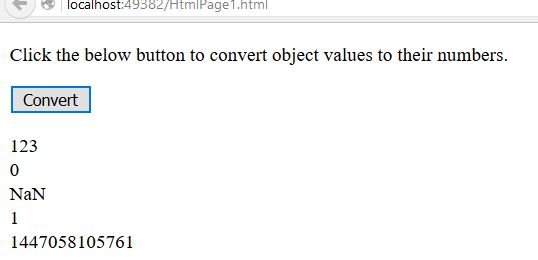# Convert object to Number in JavaScript

##### How to convert object values to their numbers in JavaScript?

By using Number() function we can convert object values to their numbers.

```<p>Click the below button to convert object values to their numbers.</p>
<input type="button" onclick="myConvert()" value="Convert">
<p id="myId"></p>

<script>
function myConvert() {
var a = "123";
var b = false;
var c = "123 456";
var d = true;
var e = new Date(); //* Returns present date and time *//

var r = Number(a) + "</br>" +
Number(b) + "</br>" +
Number(c) + "</br>" +
Number(d) + "</br>" +
Number(e);
document.getElementById("myId").innerHTML = r;
}
</script>
```

In the above code snippet we have given Id as "myId"to the second `<p>` element in the HTML code. There is a function myConvert() in the script code which is connected to the onclick of the HTML button.

We need to convert object values to their numbers, that means we need to convert the values of the variables to their numbers, for that we are using Number() function in the variable `r`.

Onclick of the button "Convert" in the HTML code fires the function myConvert() in the script code at the same time Number() function converts the variable values to their numbers and returns as output.

OUTPUTViews: 28430 | Post Order: 183